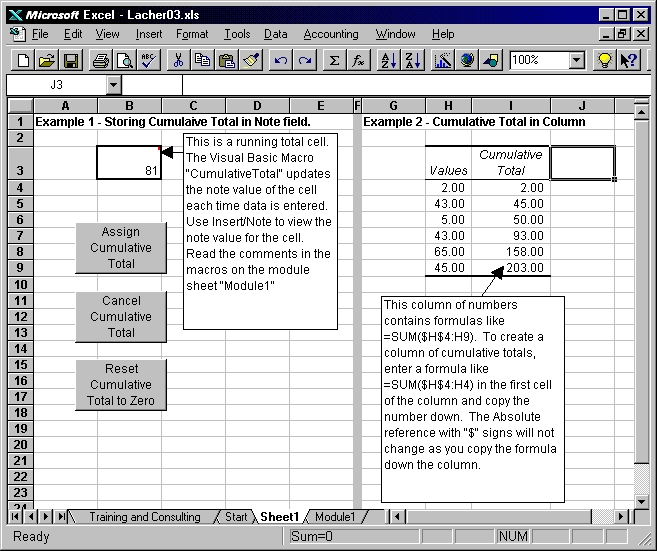## Learn How to Add Running Totals to a Worksheet

Keywords: Running Total, Cumulative Total, Note

Description: You can add a running total to a single cell or a column of data. Includes a visual basic macro and example formulas.

Tip: Excel provides several methods for creating running totals.You can use a worksheet function like =SUM(\$A\$1:A1) where the values to total are in column A and the formula is copied down column B. You can also use a visual basic macro to store a running total in the note field of a cell. When a new value is entered to the cell, the value in the note field is added to the value in the cell.

Guide to the Example: The example on Sheet1 shows two methods of storing cumulative totals - read the notes in the macro on the module sheet (Module1) to understand how to store a cumulative total in the note value of a field.